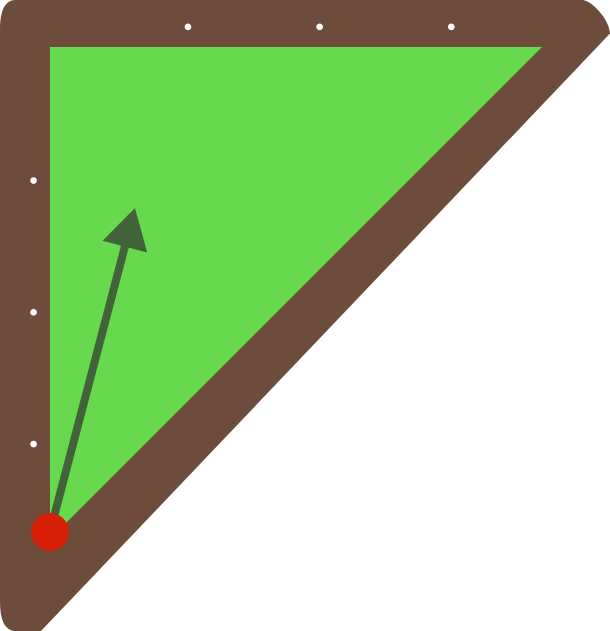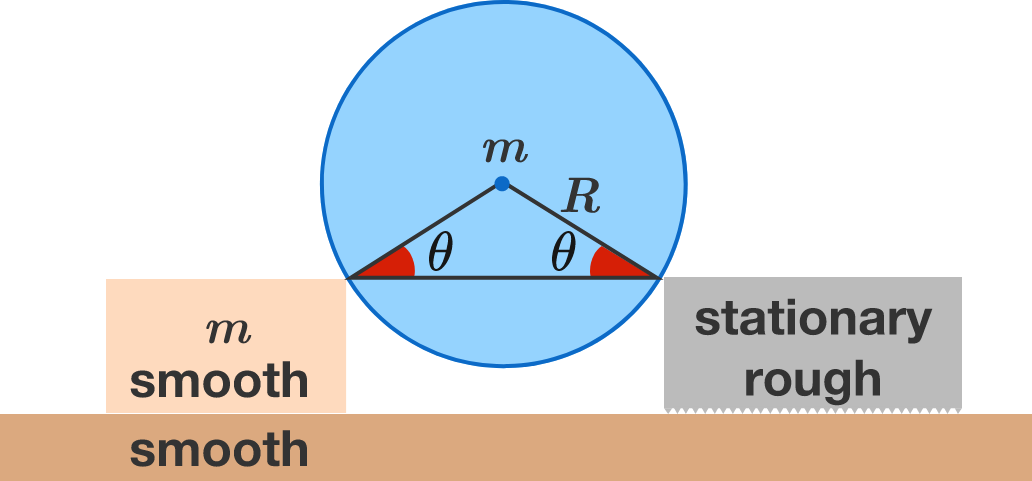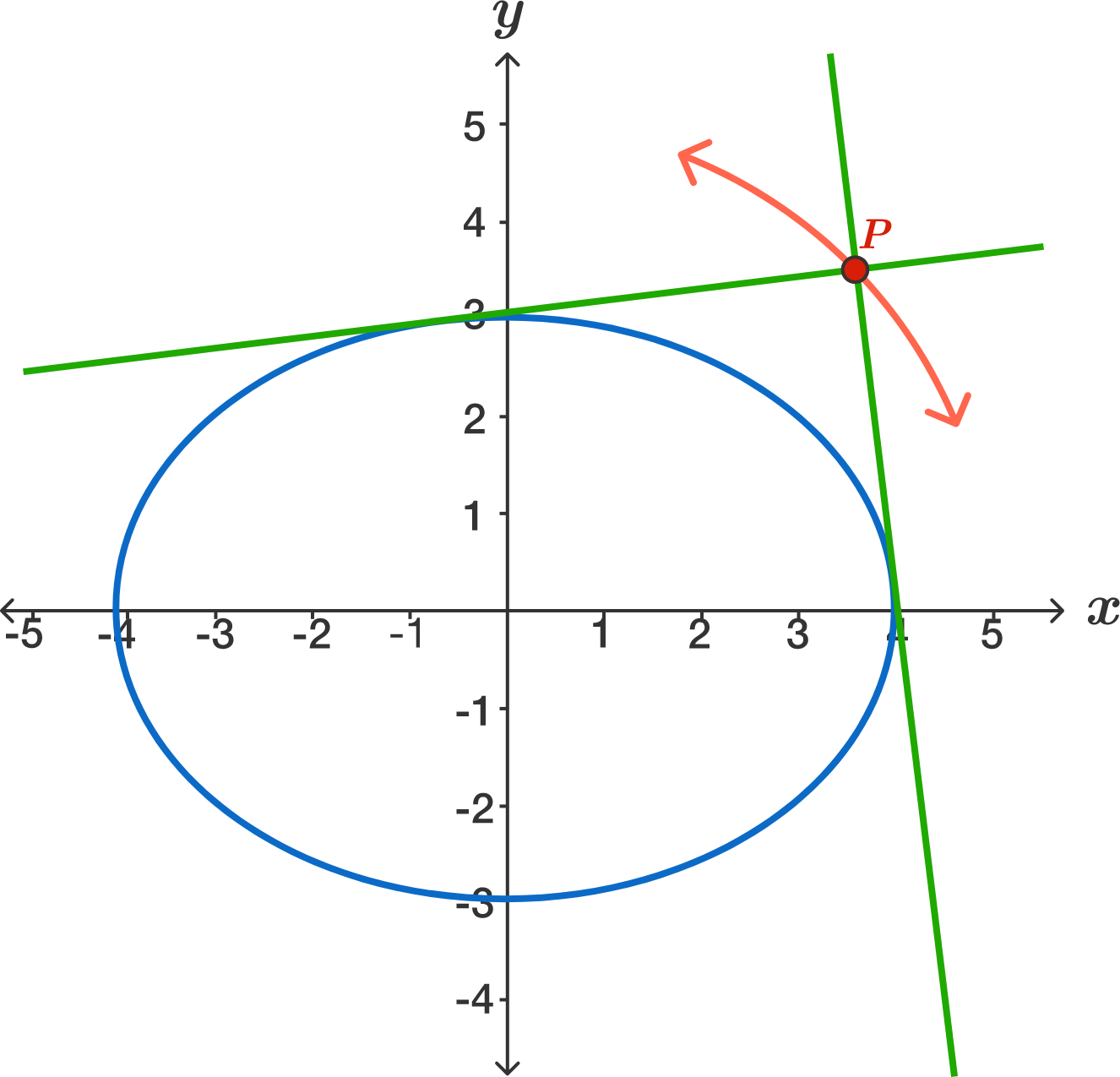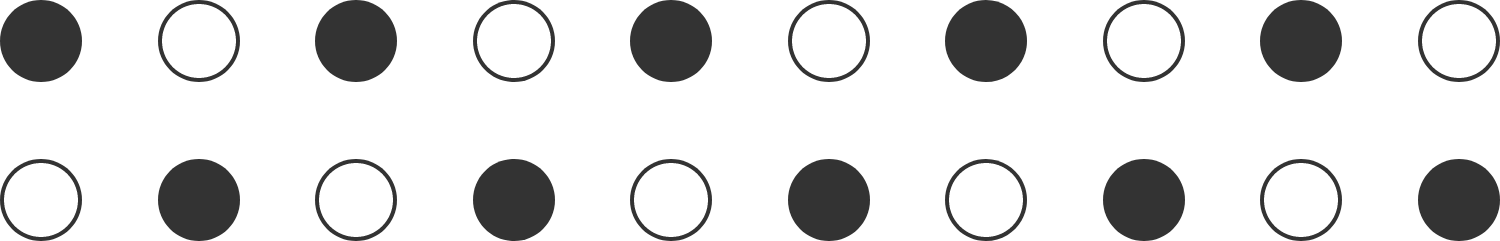# Problems of the Week

Contribute a problem

Suppose you have a triangular pool table with a ball starting at the marked vertex of the billiard table shown (shaped like an isosceles right triangle). Is it possible to hit a ball that reflects back to where it started (given any number of reflections)?Assume geometric purity: the "ball" has no mass and the "ball" and "hole" are considered single points. Also, if the ball hits a vertex point as opposed to a side, then the reflections are absorbed. (This means hitting straight up towards the right angle won't work.)

This problem is part of the Brilliant.org Open Problems Group. The end goal for each open problem is to find a solution, and maybe publish it if it's a nice enough result! You can read about the unsolved billiards problem that this one is related to here.

A solid cylinder of mass $m=5\text{ kg}$ rests on two supports of the same height, as shown. One support is rough and stationary while other is a smooth plank of the same mass placed on a smooth, horizontal surface. Initially, $\theta =37^\circ$ and there is no slipping between the cylinder and stationary support. Now, the system is released from rest.

What is the normal force $($in $\text{N})$ acting between the cylinder and the plank just after the release?

Take $g=9.8\text{ m/s}^2.$Let $f$ be a function from $\mathbb{Z}^+$ to $\mathbb{Z}^*$ such that

1. $f(1)=0$
2. $f(2n)=2f(n)+1$
3. $f(2n+1)=2f(n).$

Find the smallest value of $n$ such that $f(n)=1994$.

Notation: $\mathbb Z^+$ denotes the set of positive integers, and $\mathbb{Z}^*$ denotes the set of non-negative integers.

Below is the graph of the ellipse $\large\frac{x^2}{16}+\frac{y^2}{9}=1$ and two lines that are tangent to the ellipse and meet at right angles at a point $P.$ If we trace out the path of $P,$ its total length can be expressed as $k\pi.$ What is $k?$The diagram below shows a $2 \times 10$ grid of dots (of negligible size) that alternate between black and white. How many ways are there to draw ten line segments between the dots such that

• each white dot is connected to exactly one black dot (and vice versa),
• no segment passes through more than two dots, and
• none of the segments intersect each other?Bonus: Generalize for drawing $n$ segments on a $2 \times n$ grid, where $n$ is a positive integer.

×